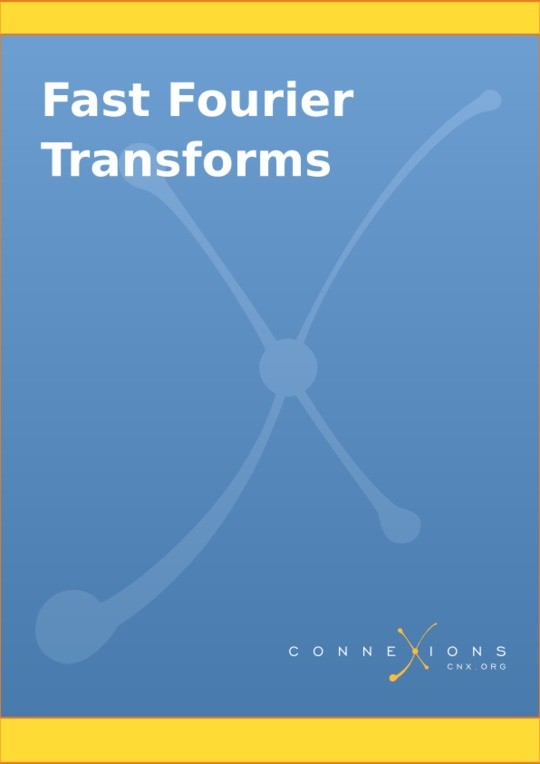Free

# Fast Fourier Transforms

By C. Burrus, Ivan Selesnick, Markus Pueschel, Steven Johnson, Matteo Frigo
Free
The publisher has enabled DRM protection, which means that you need to use the BookFusion iOS, Android or Web app to read this eBook. This eBook cannot be used outside of the BookFusion platform.
Book Description

This book uses an index map, a polynomial decomposition, an operator factorization, and a conversion to a filter to develop a very general and efficient description of fast algorithms to calculate the discrete Fourier transform (DFT). The work of Winograd is outlined, chapters by Selesnick, Pueschel, and Johnson are included, and computer programs are provided.

• Fast Fourier Transforms
• 1. Preface: Fast Fourier Transforms
• 2. Introduction: Fast Fourier Transforms
• 3. Multidimensional Index Mapping
• 4. Polynomial Description of Signals
• 5. The DFT as Convolution or Filtering
• 6. Factoring the Signal Processing Operators
• 7. Winograd's Short DFT Algorithms
• 8. DFT and FFT: An Algebraic View
• 9. The Cooley-Tukey Fast Fourier Transform Algorithm
• 10. The Prime Factor and Winograd Fourier Transform Algorithms
• 11. Implementing FFTs in Practice
• 12. Algorithms for Data with Restrictions
• 13. Convolution Algorithms
• 14. Comments: Fast Fourier Transforms
• 15. Conclusions: Fast Fourier Transforms
• 16. Appendix 1: FFT Flowgraphs
• 17. Appendix 2: Operation Counts for General Length FFT
• 18. Appendix 3: FFT Computer Programs
• 19. Appendix 4: Programs for Short FFTs
• Index